Related Articles

# Random Numbers Ecosystem in Julia – The Pseudo Side

• Last Updated : 07 Aug, 2021

When we talk of randomness in general, it’s not something that shouldn’t possess repetition, it is something that outputs data that is unpredictable

Random numbers are very essential when it comes to cryptography, MonteCarlo simulations, etc. For every time you log in to a service, make an online transaction, random numbers from the system are invoked in order to secure the process(encryption).

But our computers, specifically the CPU, are number-crunching beasts, that blindly follow the instructions that are fed to them. So they are virtually inapt to inherently pick any numbers. That’s where human intervention steps in.
Now there are two ways. The fast yet inefficient way and the slow yet near perfect way.
The former pertains to PRNGs(Pseudo Random Number Generators) and the latter to TRNGs(True Random Number Generators). Check out this link if you wish to try out some RNG algorithms.

Most operating systems(linux, windows etc.) have a built-in pool of randomness. from which random numbers for various uses are sampled. The entropy pool that consists of entropy(randomness) collected from various sources in the computer. Eg: The CPU/GPU thermals, timing of user keystokes, mouse movement, cpu scheduling and other sensors that provide unpredictable input

When random numbers are generated, the entropy of the pool is diminished since some of the information about the entropy pool is now given out. So it has to be replenished by a process called stirring
Intel’s Ivy Bridge family of processors have an integrated feature viz. secure key, which refers to a dedicated random number generator inside them, composing a special instruction set called RDRAND that returns random numbers.
Coming to RNG’s in Julia. There is a good support for Pseudo random number generation in Julia.
Inherently Julia uses the Mersenne Twister library for random number generation. Besides MersenneTwister, Julia also provides the RandomDevice type, which wraps over the operating system provided entropy.

#### rand() Function

Syntax :

`rand([rng=GLOBAL_RNG], [set..], [dimensions...])`

Picks a random element or array of random elements from the set of values specified by set, where set can be :

• an indexable collection (for example 1:9 or (‘x’, “y”, :z)),
• an AbstractDict or AbstractSet object,
• a String
• a type(eg : struct)

Example:

## Python3

 `# Generate 2 random numbers of type Int``rand(``Int``, ``2``)` `# Randomly choose a number between 3 and 5``rand((``3``, ``5``))` `# Create a 2x3 array``# that contains random floating type values``rand(Float64, (``2``, ``3``))`

Output: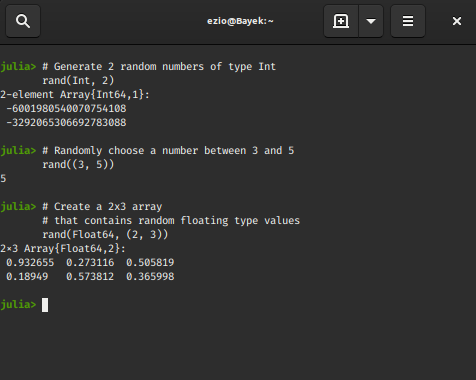#### rand!() Function

RandomNumbers.jl is a library that provides extended support for random number generation.

Syntax:

`rand!([rng=GLOBAL_RNG], Arr, [set=eltype(Arr)])`

It populates the array Arr with random values. If set is specified, the values are picked randomly from set. It simply copies the random values generated from rand() to Arr but without allocating a new array.

Example:

## Python3

 `# Import the library``using Random` `# Using the MersenneTwister rng``# Here 1234 is a seed value``rng ``=` `MersenneTwister(``1234``);` `# Create an Array containing zeros``Arr ``=` `zeros(``5``)` `# Populate Arr with random values``# Generated by our rng``rand !(rng, Arr)`

Output :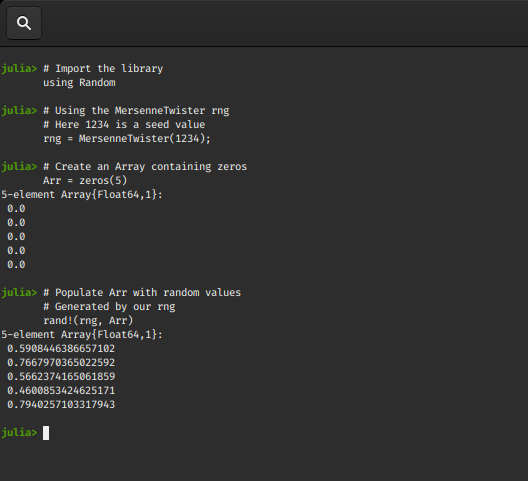#### bitrand() Function

Syntax :

`bitrand([rng=GLOBAL_RNG], [dimensions])`

It generates an Array (of type BitArray) containing random boolean values(0/1).

Example:

## Python3

 `# Use the MersenneTwister rng``rng ``=` `MersenneTwister(``1234``);` `# Generate 10 random bits``bitrand(rng, ``10``)`

Output :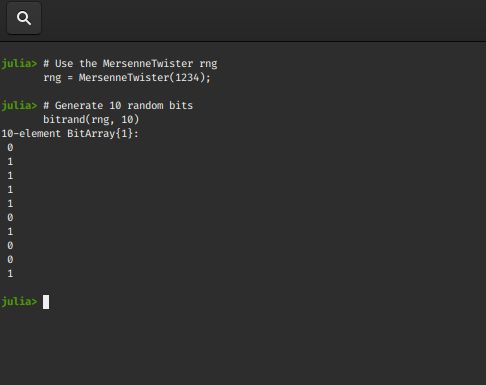#### randn() Function

Syntax:

`randn([rng=GLOBAL_RNG], [T=Float64], [dimensions])`

It generates a normally-distributed random number of type T with mean 0 and standard deviation 1. It will optionally generate an array of normally-distributed random numbers if the dimensions parameter is given.

Example:

## Python3

 `# Import the library``using Random` `# Using the rng``rng ``=` `MersenneTwister(``1234``);` `# Generate a complex number``# based normal distribution``randn(rng, ComplexF64)` `randn(rng, ComplexF32, (``2``, ``3``))`

Output: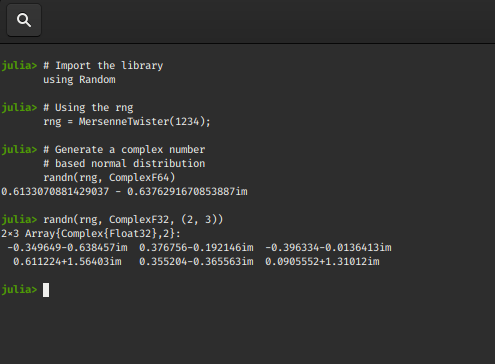#### randstring() Function

Syntax:

`randstring([rng=GLOBAL_RNG], [characters], [length])`

It creates a random string of size length, consisting of characters, which defaults to the set of upper- and lower-case letters and the digits 0-9. The optional rng argument specifies a random number generator.

Example:

## Python3

 `# Import the library``using Random` `# Seed the rng``Random.seed !(``0``);` `# Return an random string``randstring()` `# Return a random string``# of size 6, made from  characters a-z``randstring(MersenneTwister(``0``), ``'a'``:``'z'``, ``6``)` `# To generate random DNA sequence``randstring(``"ACGT"``)`

Output: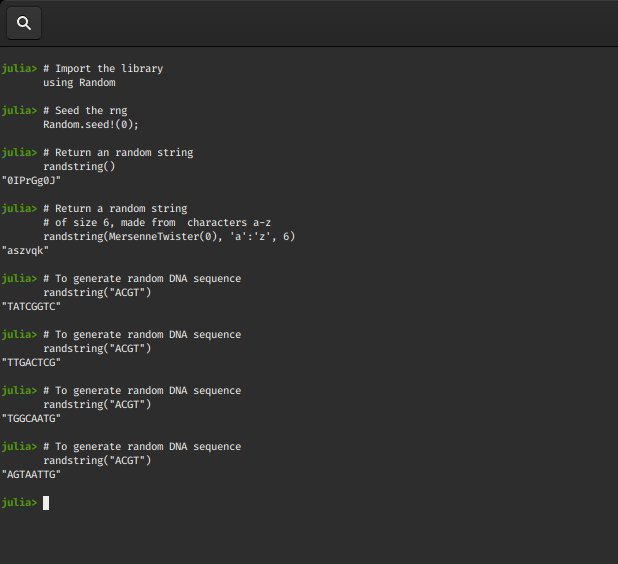#### randperm() Function

Syntax:

`randperm([rng=GLOBAL_RNG], n::Integer)`

Construct a random permutation of length n.

Examples:

## Python3

 `# Import the library``using Random` `# Using the rng``rng ``=` `MersenneTwister(``1234``);` `# Generate a random permutation of size 4``randperm(rng, ``4``)`  `# Store the generated values in Arr``randperm !(rng, Vector{``Int``}(undef, ``4``))`

Output: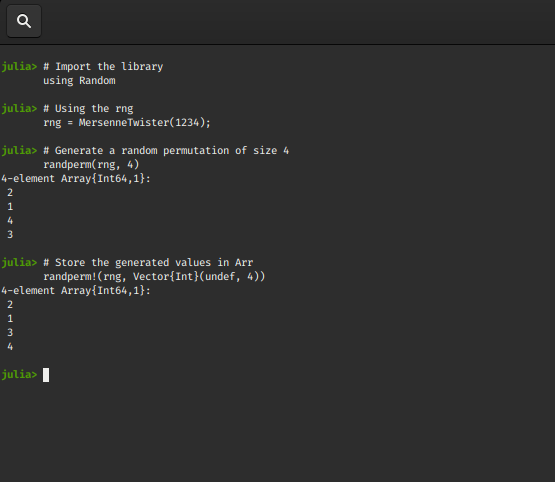#### RandomDevice() Function

Syntax :

`RandomDevice()`

Creates a RandomDevice RNG object that generates a stream of random numbers for which the entropy is obtained from the operating system

#### Sampler() function

Syntax:

`Sampler(rng, x, repetition)`

It returns a sampler object that can be used to generate random values from rng for x
When Sampler(rng, x, repetition), rand(rng, sp) is used to draw random values, repetition can be Val(1) or Val(Inf).

Example :

## Python3

 `# import the library``using Random` `# Define the rng``rng ``=` `RandomDevice()` `# Define the sampler``s ``=` `Random.Sampler(rng, Val(``1``))`

Output: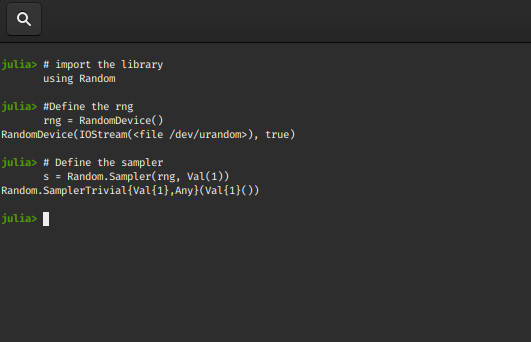### Generating values from a type

For a type T, it’s assumed that if rand(T) is defined, an object of type T will be produced. The default sampler for types is SamplerType.

Syntax:

`rand(rng::AbstractRNG, ::Random.SamplerType{T}) `

Defines random generation for values of type T

This is particularly useful in cases like the classical example of a dice that gives a random number.

## Python3

 `# declare a dice type``struct Dice``    ``n::``Int` `# number on the dice``end` `# Define the rand function to generate``# random numbers between 1-6``Random.rand(rng::AbstractRNG, ::Random.SamplerType{Dice}) ``=` `Dice(rand(rng, ``1``:``6``))` `# generate random number of sides``# based on the default rng``rand(Dice)` `# generate random number of sides``# based on the MersenneTwister rng``rand(MersenneTwister(``0``), Dice)`

Output: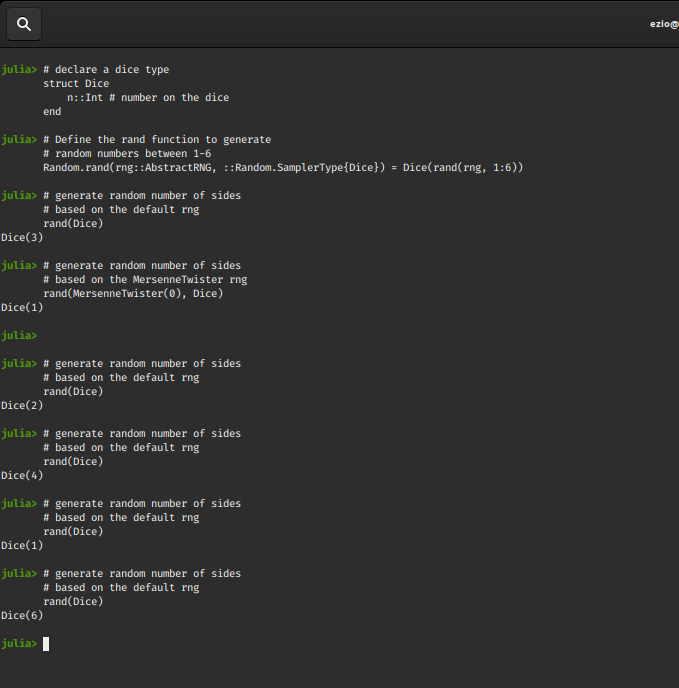My Personal Notes arrow_drop_up